# matplotlib.pyplot.gca#

matplotlib.pyplot.gca()[source]#

Get the current Axes.

If there is currently no Axes on this Figure, a new one is created using `Figure.add_subplot`. (To test whether there is currently an Axes on a Figure, check whether `figure.axes` is empty. To test whether there is currently a Figure on the pyplot figure stack, check whether `pyplot.get_fignums()` is empty.)

## Examples using `matplotlib.pyplot.gca`#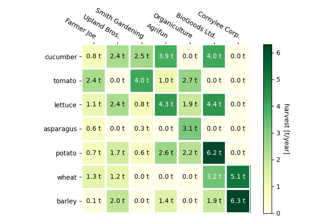Creating annotated heatmaps

Creating annotated heatmaps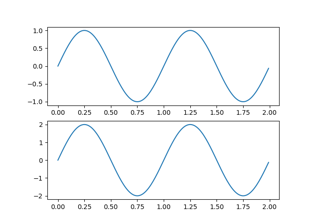Managing multiple figures in pyplot

Managing multiple figures in pyplot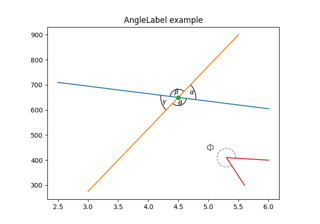Scale invariant angle label

Scale invariant angle label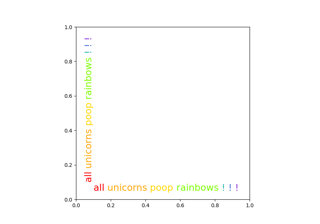Rainbow text

Rainbow text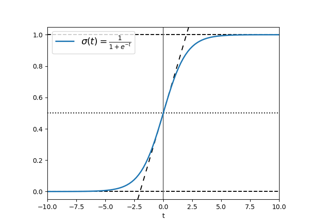Infinite lines

Infinite lines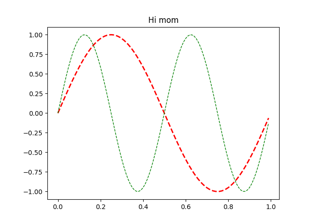Set and get properties

Set and get properties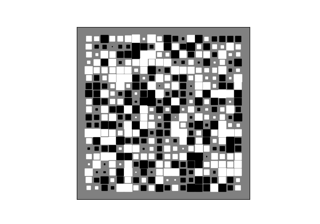Hinton diagrams

Hinton diagrams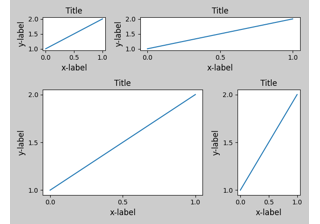Tight Layout guide

Tight Layout guide